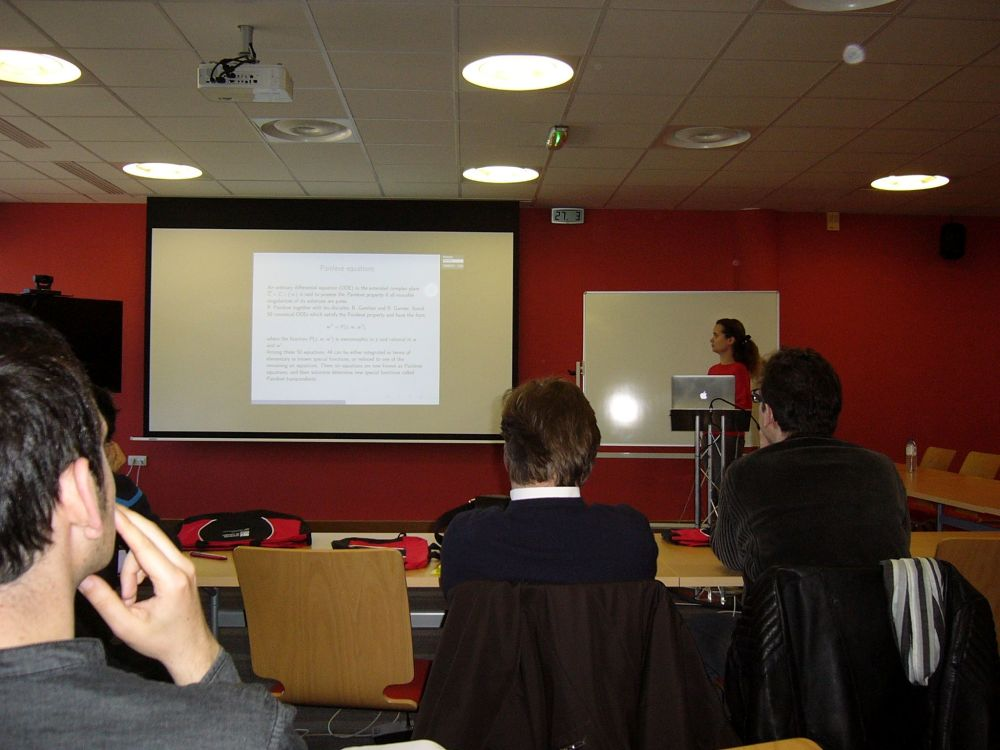• A
• A
• A
• ABC
• ABC
• ABC
• А
• А
• А
• А
• А
Regular version of the site

# 10th International Conference on Functional Equations

On March 27-29, 2017 the tenth annual conference ‘Functional Equations in Limoges 2017’ was held at the Faculty of Science and Technology at the University of Limoges (France).The following topics were among those covered during the conference: differential equations, difference equations, partial differential equations, integrating dynamical systems. Mathematicians from Austria, Germany, Colombia, Poland, Russia, the USA and France took part in the event.

Anastasia Parusnikova, Associate Professor at MIEM School of Applied Mathematics, presented the report ‘On asymptotic expansions of solutions to the third Painlevé equation in the neighborhood of infinity’. The paper presents a family of values for the parameters of the third Painlevé equation such that Puiseux series formally satisfying this equation - series of z^{2/3} - are series of exact Gevrey order one. The researcher proves the divergence of these series and provides analytic functions which are approximated by them in sectors with the vertices at infinity.